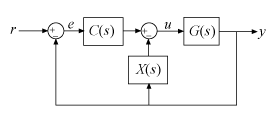Documentation

## Decompose a 2-DOF PID Controller into SISO Components

This example shows how to extract SISO control components from a 2-DOF PID controller in each of the feedforward, feedback, and filter configurations. The example compares the closed-loop systems in all configurations to confirm that they are all equivalent.

Obtain a 2-DOF PID controller. For this example, create a plant model, and tune a 2-DOF PID controller for it.

```G = tf(1,[1 0.5 0.1]); C2 = pidtune(G,'pidf2',1.5);```

`C2` is a `pid2` controller object. The control architecture for `C2` is as shown in the following illustration.This control system can be equivalently represented in several other architectures that use only SISO components. In the feedforward configuration, the 2-DOF controller is represented as a SISO PID controller and a feedforward compensator.Decompose `C2` into SISO control components using the feedforward configuration.

`[Cff,Xff] = getComponents(C2,'feedforward')`
```Cff = 1 s Kp + Ki * --- + Kd * -------- s Tf*s+1 with Kp = 1.12, Ki = 0.23, Kd = 1.3, Tf = 0.122 Continuous-time PIDF controller in parallel form. Xff = -10.898 (s+0.2838) ------------------ (s+8.181) Continuous-time zero/pole/gain model. ```

This command returns the SISO PID controller `Cff` as a `pid` object. The feedforward compensator `X` is returned as a `zpk` object.

Construct the closed-loop system for the feedforward configuration.

`Tff = G*(Cff+Xff)*feedback(1,G*Cff);`

In the feedback configuration, the 2-DOF controller is represented as a SISO PID controller and an additional feedback compensator.Decompose `C2` using the feedback configuration and construct that closed-loop system.

```[Cfb, Xfb] = getComponents(C2,'feedback'); Tfb = G*Cfb*feedback(1,G*(Cfb+Xfb));```

In the filter configuration, the 2-DOF controller is represented as a SISO PID controller and prefilter on the reference signal.Decompose `C2` using the filter configuration. Construct that closed-loop system as well.

```[Cfr, Xfr] = getComponents(C2,'filter'); Tfr = Xfr*feedback(G*Cfr,1);```

Construct the closed-loop system for the original 2-DOF controller, `C2`. To do so, convert `C2` to a two-input, one-output transfer function, and use array indexing to access the channels.

```Ctf = tf(C2); Cr = Ctf(1); Cy = Ctf(2); T = Cr*feedback(G,Cy,+1);```

Compare the step responses of all the closed-loop systems.

```stepplot(T,Tff,Tfb,Tfr) legend('2-DOF','feedforward','feedback','filter','Location','Southeast')```The plots coincide, demonstrating that all the systems are equivalent.

Using a 2-DOF PID controller can yield improved performance compared to a 1-DOF controller. For more information, see Tune 2-DOF PID Controller (Command Line).